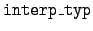Next: File formats Up: Various identifiers Previous: Kinematics types   Contents

# Interpolation

The interpolation code interp_typ used in the ENDL system specifies the type of interpolation to be used for the data on energy and cross section. Only the values= 0 and 5 are used.= 0:
Linear-linear interpolation. This is used for all but incident gammas.= 1:
Piecewise constant (a histogram).= 2:
Linear-linear interpolation.= 3:= 4:= 5: